# Eigenvalue and Eigenvector in Linear Algebra Lesson Plans Chapter Exam

Exam Instructions:

Choose your answers to the questions and click 'Next' to see the next set of questions. You can skip questions if you would like and come back to them later with the yellow "Go To First Skipped Question" button. When you have completed the practice exam, a green submit button will appear. Click it to see your results. Good luck!

### Page 1

#### Question 2 2. Find the eigenvectors associated with the eigenvalue of 4 for the following matrix.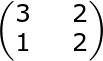#### Question 3 3. In order to find a matrix's eigenvectors, we solve the following equation. Which of this equation's variables represents the eigenvectors?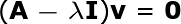#### Question 4 4. Find the eigenvectors associated with the eigenvalue of 0 for the following matrix.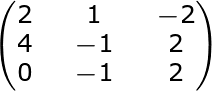#### Question 5 5. Find the eigenvectors associated with the eigenvalue of 4 for the following matrix.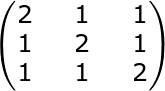### Page 2

#### Question 6 6. The matrix, A, is a _____.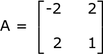#### Question 7 7. If the eigenvector of a matrix is the vector, v1, shown below, then the length of v1 is _____.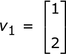#### Question 8 8. To diagonalize a matrix, A, we calculate Pt AP. For the normalized eigenvectors, u1 and u2, the matrix, P, is given by _____.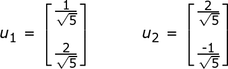#### Question 9 9. For the eigenvector, v, the normalized eigenvector, u, is _____.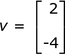#### Question 10 10. To diagonalize a symmetric matrix, A, we calculate Pt AP. The result is the matrix, D, where D is the diagonal matrix shown. The eigenvalues of A are _____.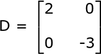### Page 3

#### Question 11 11. Find all the eigenvalues of the following 2x2 matrix (A).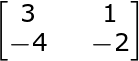#### Question 13 13. Find all the eigenvalues for the following 2x2 matrix (A).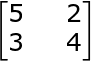#### Question 14 14. Find all the eigenvalues for the following 3x3 matrix (A).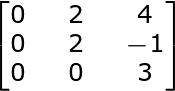### Page 5

#### Question 21 21. One of the eigenvalues of the following matrix is -2. Find the corresponding eigenvector where we put 1 in for the first parameter of the vector.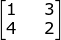#### Question 23 23. Find the eigenvalues of the following matrix.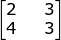#### Question 24 24. For any real number x, the following is an eigenvector of a matrix A. Find its unit eigenvector.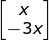#### Eigenvalue and Eigenvector in Linear Algebra Lesson Plans Chapter Exam Instructions

Choose your answers to the questions and click 'Next' to see the next set of questions. You can skip questions if you would like and come back to them later with the yellow "Go To First Skipped Question" button. When you have completed the practice exam, a green submit button will appear. Click it to see your results. Good luck!

Support